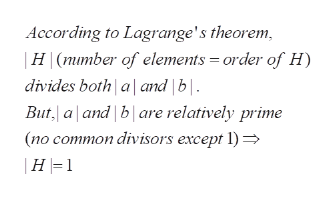# 64. Let a and b belong to a group. If lal and lbl are relatively prime,show that (a) n (b) = {e}.

Question
13 views

64help_outlineImage Transcriptionclose64. Let a and b belong to a group. If lal and lbl are relatively prime, show that (a) n (b) = {e}. fullscreen
check_circle

Step 1

Under the given conditions, to show that the cyclic groups generated by a and b have only common element, namely the identity

Step 2

Note that H is a subgroup , as it is the intersection of two subgroups <a> and <b>

Step 3

conclude first that <a> intersection <b> ...help_outlineImage TranscriptioncloseAccording to Lagrange's theorem |H (number of elements order of H) divides both aand b| But, aland b are relatively prime (no common divisors except 1) |H 1 fullscreen

### Want to see the full answer?

See Solution

#### Want to see this answer and more?

Solutions are written by subject experts who are available 24/7. Questions are typically answered within 1 hour.*

See Solution
*Response times may vary by subject and question.
Tagged in

### Math